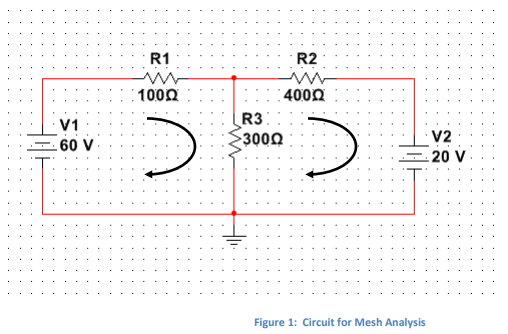# Module 5 Lab: Mesh and Nodal Analysis

15.00 \$

Category:
Click Category Button to View Your Next Assignment | Homework

You'll get a: . ` pdf` file solution, download link after Payment

## Description

The purpose of this lab is to investigate the technique of Mesh and Nodal Analysis to analyze electrical circuits.

1. Mesh Analysis Calculations

1.1 Consider the circuit shown in Figure 1.1.2 Write the equations for the two mesh currents.

1.3 Solve the equations and find the values of the following currents:

Calculated Values

I out 60 V Source I R1 I R2 I R3 I in 20 V Source

1.4 Build the circuit in Multisim and measure the current values. Complete the table below.

Measured Values

I out 60 V Source I R1 I R2 I R3 I in 20 V Source

1.5 Are these values similar? Explain your results.

2. Nodal Analysis Calculations

2.1 Consider the circuit shown in Figure 2.2.2 Write the Nodal Equations for this circuit.

ELEC 152 – Module 5 Laboratory – Page 3

2.3 Solve the Nodal Equations in order to find the two unknown Nodal Voltages.

V1 =
V2 =

2.4 We will now proceed to building the circuit from Figure 2. This circuit uses a new element, the Current Source. Figure 3 below shows the location of the current source in Multisim. After the source has been placed,
double clicking it will allow changing some of its parameters, in particular the value of the current.2.5 Build the circuit in Multisim and measure the values of the 2 Nodal Voltages:
V1 =
V2 =2.6 Compare these results with the expected results from Section 2.3. Are they similar? Comment on your results.